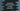# Add an element to an array at a specific position in JavaScript## How to add an element to an array at a specific position in JavaScript:

Let’s learn how to add an element at a specific position to a JavaScript array. For example, let’s say you have this array:

``[1, 2, 3, 5, 6]``

And, you want to add 4 to the fourth position and change this array to this:

``[1, 2, 3, 4, 5, 6]``

We have added 4 at index 3. The index starts from 0 in a JavaScript array and ends at length - 1, where length is the length of the array or the total number of items in that array.

There is an inbuilt method available to do this operation. This method is called splice(). In this post, we will learn the definition of splice and how we can use it to add an element to an array at any given index.

### Definition of splice:

splice or Array.prototype.splice() method is defined as like below:

``splice(start, count, items)``

This method can be used to do in place modification of an array. We can:

• Add new elements to an array
• Delete elements from an array
• Modify elements of an array

Here,

• start is the starting index for the process. If its value is more than the length of the array, it will only add elements to the array. For a negative value, it will start that many items from the end of the array. If it is negative infinity, it starts from the 0th index.
• count is an optional value. This is the number of items to remove from the start index. If we don’t provide its value, it will remove all elements from start to end of the array.
• items are items to add to the array starting from start. This is an optional value. If we don’t provide any element, it will only remove elements from that array.

### Return value of splice:

splice method returns all deleted elements in an array. If no element is deleted, it returns an empty array.

### How to add an element at specific position using splice:

We can use splice to add single or multiple elements at a specific position of an array.

• We can provide the start index as the position to insert element
• count is 0 because we don’t want to remove any item.
• The items to add as the third, fourth… parameters.

### Example program:

``````var arr = [1,2,3,4,5];

console.log(arr);
arr.splice(2, 0, 10);
console.log(arr);``````

This method is adding 10 at index 2 of arr.

If you run this program, it will print:

``````[ 1, 2, 3, 4, 5 ]
[ 1, 2, 10, 3, 4, 5 ]``````

### Example to add multiple items:

Let’s try to add multiple items to an array:

``````var arr = [1,2,3,4,5];

console.log(arr);
arr.splice(2, 0, 10, 20, 30);
console.log(arr);``````

It will add 10, 20 and 30 from index 2 in arr array:

``````[ 1, 2, 3, 4, 5 ]
[1, 2, 10, 20, 30, 3,  4,  5]``````

### Example with negative index:

We can also use a negative index as the index position. For example:

``````var arr = [1,2,3,4,5];

console.log(arr);
arr.splice(-2, 0, 20);
console.log(arr);``````

It will add one element after the second element from the end.

``````[ 1, 2, 3, 4, 5 ]
[ 1, 2, 3, 20, 4, 5 ]``````

### If start is more than the length of the array:

If the value of start is larger than the length of the array, it will add the item to the end of the array. For example,

``````var arr = [1,2,3,4,5];

console.log(arr);
arr.splice(15, 0, 10);
console.log(arr);``````

It will print:

``````[ 1, 2, 3, 4, 5 ]
[ 1, 2, 3, 4, 5, 10 ]``````